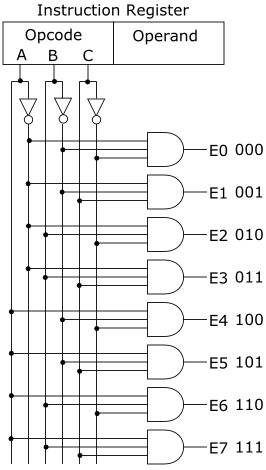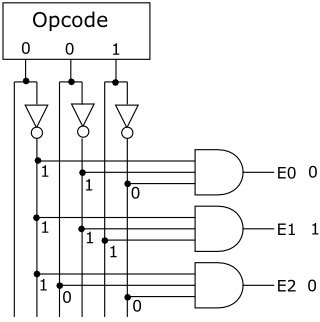#Basic Decoder Circuitry by Stephen Bucaro

A microprocessors is a circuit that reads and execute program instructions. The instructions are in binary coded form, called opcodes. Many instructions involve data that an operation is to be performed on. That data may be part of the instruction, or may be "pointed to" by the instruction. An important part of a microprocessor is the circuit that decodes the instructions.

A decoder is a circuit that converts an opcode into a signal or a set of signals that are used to control the circuitry of the microprocessor to enable it to perform the instruction defined by the opcode. A decoder may convert an opcode into an Enable input to control the circuitry.

Shown below is an example of a simple decoder that decodes a 3-bit opcode into one of eight control signals.The input to the decoder is a 3-bit opcode, A, B, C. The decoder has three inverters that provide the compliments to the three inputs _A, _B, _C. AND gates are connected to specific signals such that only when all of its inputs are binary 1, will the output of the AND gate be 1. That output will be an Enable input to control the circuitry that performs that opcode's function. Lets focus in on a specific section of the decoder.The opcode in the circuit shown above is 001. Note that that the AND gate with output E1 is the only gate with 1's on all three inputs. This causes the gates output to be 1. All other gates have at least one 0 on an input, causing their outputs to be 0. The E1 output could be the control signal to load a register, or it could be the control signal to cause an ALU to add or subtract.

This describes a basic 3-bit decoder. The Intel Pentium microprocessor uses 32-bit instructions, and many of it's opcodes generate multiple enable signals, so you can imagine the complexity of a Pentium's decoder.